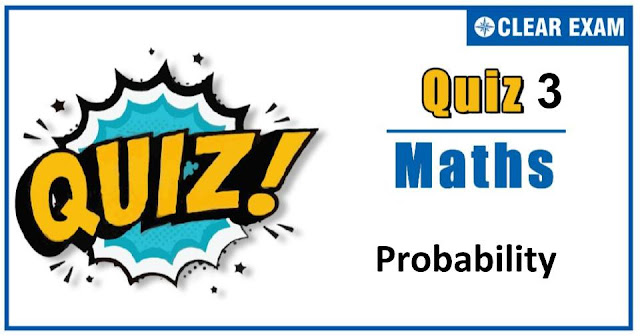## [LATEST]\$type=sticky\$show=home\$rm=0\$va=0\$count=4\$va=0

As per analysis for previous years, it has been observed that students preparing for JEE MAINS find Mathematics out of all the sections to be complex to handle and the majority of them are not able to comprehend the reason behind it. This problem arises especially because these aspirants appearing for the examination are more inclined to have a keen interest in Mathematics due to their ENGINEERING background.

Furthermore, sections such as Mathematics are dominantly based on theories, laws, numerical in comparison to a section of Engineering which is more of fact-based, Physics, and includes substantial explanations. By using the table given below, you easily and directly access to the topics and respective links of MCQs. Moreover, to make learning smooth and efficient, all the questions come with their supportive solutions to make utilization of time even more productive. Students will be covered for all their studies as the topics are available from basics to even the most advanced.

Q1. In tossing of a coin (m+n)(m>n) times, the probability of coming consecutive heads at least m times is
•  (n+2)/2(m+1)
•  (m-n)/2(m+n)
•  (m+n)/2(m+n)
•  (mn)/2(m+n)
Solution
(a) Let 'H'denote the head and any of them come is 'A'.Suppose the sequence of m consecutive heads start with first throw, then P[(HH….m times)(AA…n times)] =(1/2.1/2….m times)(1..1.….n times) =(1/2)m Now, suppose the sequence of m consecutive heads start with second throw, the first must be a tail, ∴P[T(H.H…m times)(A.A…n-1 times)] =1/2.1/2m ×(1)(n-1)=1/2(m+1) Similarly, as above ∴Required probability =1/2m +(1/2(m+1) +1/2(m+1) +⋯n times) =1/2m +n/2(m+1) =(n+2)/2(m+1)

Q2.An urn contains nine balls of which three are red, four are blue and two are green. Three balls are drawn at random without replacement from the urn. The probability that the three balls have different colours, is
•  1/3
•  2/7
•  1/21
•  2/23
Solution
(b) Total number of cases= 9C3=84 Number of favourable cases= 3C1.4C1.2C1=24 ∴ P=24/84=2/7
Q3.  There are 9999 tickets bearing numbers 0001, 0002,….,9999. If one ticket is selected from these tickets at random, the probability that the number on the ticket will consists of all different digits, is
•   5040/9999
•  5000/9999
•  5030/9999
•  None of these
Solution
(a) Total number of cases = 9999 Favourable cases =10×9×8×7=5040 ∴ Probability =5040/9999

Q4. A man takes a step forward with probability 0.4 and back-ward with probability 0.6. The probability that at the end of eleven steps he is one step away from the starting point is
•  11C6(0.24)5
•  11C6(0.4)6 (0.6)5
•  11C6(0.6)6 (0.4)5
•  None of these
Solution
(a) The man will be one step away from the starting point if (i) either he is one step ahead or (ii) one step behind the starting point ∴ Required probability =P(i)+P(ii) The man will be one step ahead at the end of eleven steps if he moves six steps forward and five steps backward. The probability of this even is 11C6(0.4)6 (0.6)5 The man will be one step behind at the end of eleven steps if he moves six steps backward and five steps forward The probability of this event is 11C6(0.6)6 (0.4)5 Hence, required probability = 11C6(0.4)6 (0.6)5+ 11C6(0.6)6 (0.4)5 = 11C6(0.4)5 (0.6)5 (0.4+0.6)= 11C6(0.24)5

Q5.A and B are two independent event such that P(A)=1/5,P(A∪B)=7/10. Then, P(B')=
•  3/8
•  2/7
•  7/9
•  None of these
Q6. Four boys and three girls stand in a queue for an interview, probability that they will in alternate position, is
•  1/34
•  1/35
• 1/17
•  1/68
Solution
(b) First we fix the position of a girl between one place. Girls can be arranged in 3! ways. There are four alternate places to be left. Therefore four boys occupy the place in 4! ways. ∴ The favourable cases =4!×3! Hence, the required probability =(4!×3!)/7!=6/(7×6×5)=1/35

Q7.The probability that the 6th day of a randomly chosen month of a year is a Sunday, is
•  1/12
•  1/17
•  1/84
•  None of these
Solution
(c) Any month out of 12 months can be chosen with probability 1/12 There are 7possiblle ways, in which the month can start and it will be a Sunday on 6th day, if the first day of the month is Tuesday, whose probability is 1/7 ∴ Required probability is 1/7 ∴ Required probability=1/12×1/7=1/84

Q8.Twelve tickets are numbered from 1 to 12. One ticket is drawn at random, then the probability of the number to be divisible by 2 or 3, is
•  2/3
•  7/12
•  5/6
•  3/4
Solution
(a) Let E be the event of numbers to be divisible by 2 or 3 ∴ E={2,3,4,6,8,9,10,12} ⇒ n(E)=8 and n(S)=12 Hence,required probability=n(E)/n(S) =8/12=2/3

Q9.Three identical dice are rolled. The probability that the same number will appear on each of them, is
•  1/6
•  1/36
•  1/18
•  3/28
Solution
(b) Let Ai denote an event of getting number i(i=1,2,…,6) on each die. Then, Ai,i=1,2,…,6 are mutually exclusive events ∴ Required probability =P(A1 )+P(A2 )+⋯+P(A6) …(i) Now, P(Ai )= Probability of getting number i on each die ⇒P(Ai )=1/6×1/6×1/6=1/216 So, required probability =6/216=1/36 [From (i)] ALITER We have, Total number of elementary events =6×6×6=216 Same number can be obtained on each die in one of the following ways: (1,1,1),(2,2,2),(3,3,3),(4,4,4),(5,5,5),(6,6,6) Favourable number of elementary events =6 Hence, required probability =6/216=1/36

Q10. A box contains 100 bulbs out of which 10 are defective. A sample of 5 bulbs is drawn. The probability that none is defective, is
•  (1/10)5
•  (1/2)5
•  (9/10)5
• 9/10
Solution
(c) Let X be the number of defective bulbs in a sample of 5 bulbs. Probability that a bulb is defective =p=10/100=1/10 Then, P(X=r)= 5Cr (1/10)r (9/10)(5-r) ∴ Required probability =P(X=0)= 5 C0 (1/10)0 (9/10)5=(9/10)5## Want to know more

Want to Know More
Please fill in the details below:

## Latest NEET Articles\$type=three\$c=3\$author=hide\$comment=hide\$rm=hide\$date=hide\$snippet=hide

Name

ltr
item
Best IIT JEE Coaching Institute in Delhi | NEET Coaching Institute in Delhi: Probability-quiz-3
Probability-quiz-3